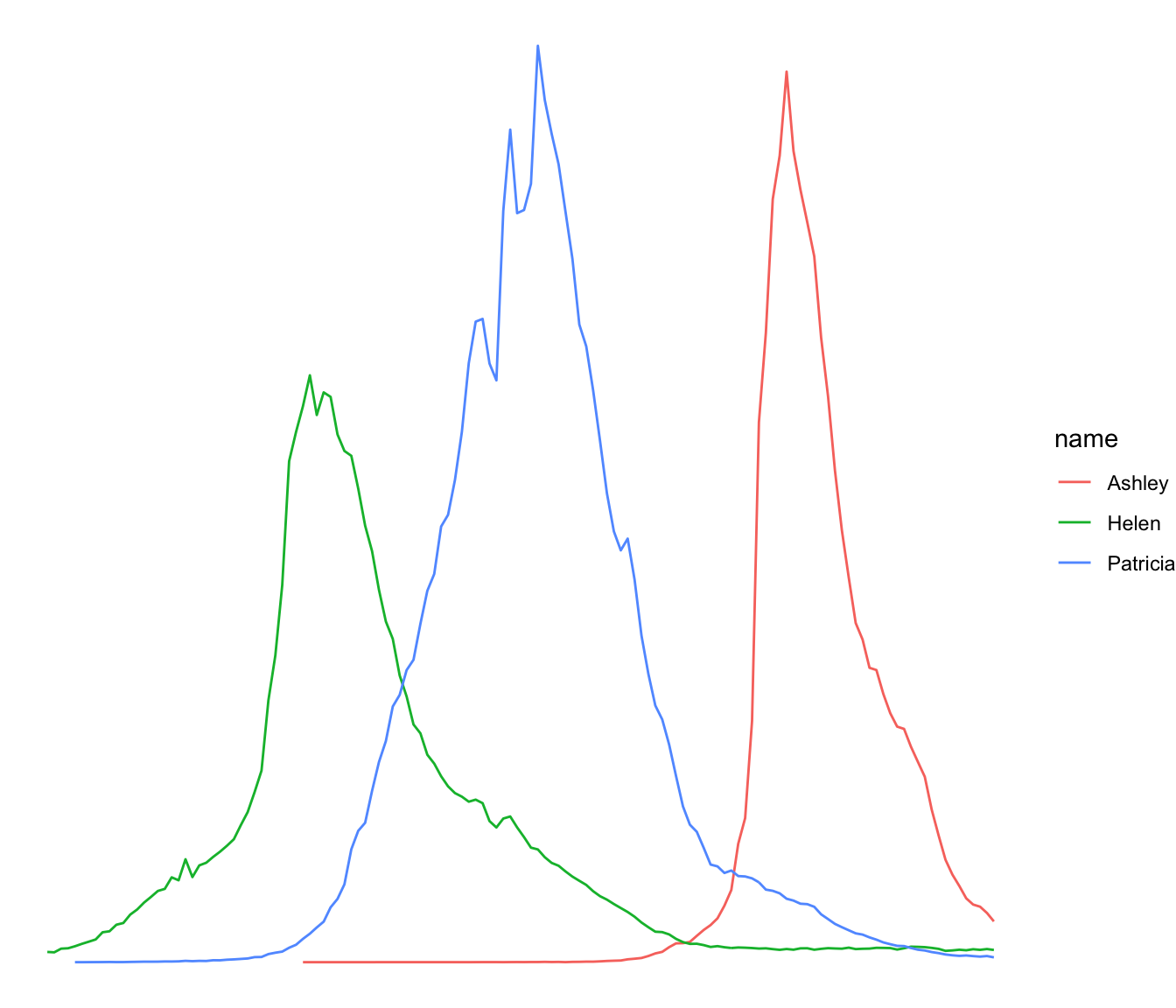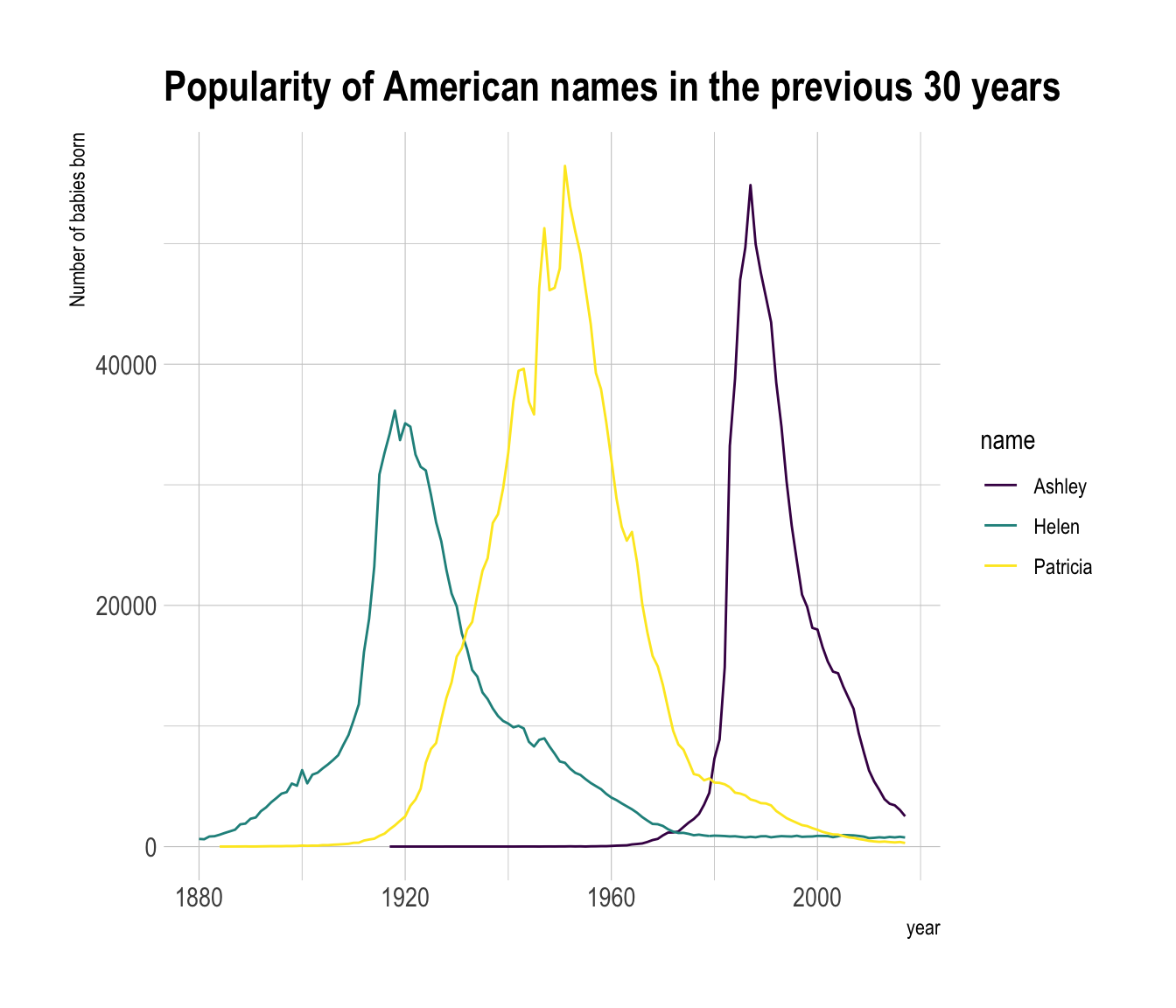# Multi groups line chart with ggplot2

This post explains how to build a line chart that represents several groups with ggplot2. It provides several examples with explanation and reproducible code.

# Basic version

If you’re not familiar with the `geom_line()` function, you should probably have a look to the most basic line chart first.

Here, the input data frame is composed by 3 columns:

• An ordered numeric variable for the X axis
• Another numeric variable for the Y axis
• A categorical variable that specify the group of the observation

The idea is to draw one line per group. This is doable by specifying a different color to each group with the `color` argument of `ggplot2`.``````# Libraries
library(ggplot2)
library(babynames) # provide the dataset: a dataframe called babynames
library(dplyr)

# Keep only 3 names
don <- babynames %>%
filter(name %in% c("Ashley", "Patricia", "Helen")) %>%
filter(sex=="F")

# Plot
don %>%
ggplot( aes(x=year, y=n, group=name, color=name)) +
geom_line()``````

# Customize the grouped line chart

Several options are available to customize the line chart appearance:

• Add a title with `ggtitle()`.
• Change line style with arguments like `shape`, `size`, `color` and more.
• Use the `viridis` package to get a nice color palette.
• Custom the general theme with the `theme_ipsum()` function of the `hrbrthemes` package.

More generally, visit the [ggplot2 section] for more ggplot2 related stuff.``````# Libraries
library(ggplot2)
library(babynames) # provide the dataset: a dataframe called babynames
library(dplyr)
library(hrbrthemes)
library(viridis)

# Keep only 3 names
don <- babynames %>%
filter(name %in% c("Ashley", "Patricia", "Helen")) %>%
filter(sex=="F")

# Plot
don %>%
ggplot( aes(x=year, y=n, group=name, color=name)) +
geom_line() +
scale_color_viridis(discrete = TRUE) +
ggtitle("Popularity of American names in the previous 30 years") +
theme_ipsum() +
ylab("Number of babies born")``````